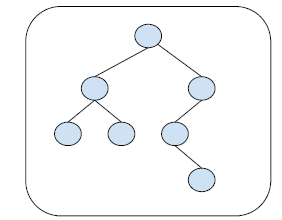# Find the Deepest Node in a Binary Tree in C++

In this problem, we are given a binary tree. Our task is to find the Deepest Node in a Binary Tree.

Binary Tree is a special data structure used for data storage purposes. A binary tree has a special condition that each node can have a maximum of two children.

Deepest node in a binary tree is the node which is at the maximum possible height in the tree.

Let's take an example to understand the problem,

Input :Output : 8

## Solution Approach

There can be multiple ways to solve this problem, as you need to find the height and traverse the tree to the last node at the height and return it. All solutions will lie on this principle only. Here, we have discussed some promising and optimised solutions.

A simple solution to the problem is using the inorder traversal of the tree and maintain a level mark, if the current level is greater than maxLevel. We will initialise the node as deepestNode. After all nodes of the tree are traversed, we will return the deepestNode. For the traversal of the tree, we will use recursion.

## Example

Program to illustrate the working of our solution

#include <iostream>
using namespace std;
struct Node{
int data;
struct Node *left, *right;
};
Node *newNode(int data){
Node *temp = new Node;
temp->data = data;
temp->left = temp->right = NULL;
return temp;
}
void findDeepestNodeRec(Node *root, int currentLevel, int &maxLevel, int &deepestNode){
if (root != NULL){
findDeepestNodeRec(root->left, ++currentLevel, maxLevel, deepestNode);
if (currentLevel > maxLevel){
deepestNode = root->data;
maxLevel = currentLevel;
}
findDeepestNodeRec(root->right, currentLevel, maxLevel, deepestNode);
}
}
int findDeepestNodeBT(Node *root){
int deepestNode = 0;
int maxLevel = 0;
findDeepestNodeRec(root, 0, maxLevel, deepestNode);
return deepestNode;
}
int main(){
Node* root = newNode(3);
root->left = newNode(5);
root->right = newNode(4);
root->left->left = newNode(1);
root->left->right = newNode(9);
root->right->left = newNode(6);
root->right->left->right = newNode(8);
cout<<"The deepest node of the given binary tree is "<<findDeepestNodeBT(root);
return 0;
}

## Output

The deepest node of the given binary tree is 8


Another approach to solve the problem is by finding the height of the given tree. And then return the node which is at the level equal to height of the tree.

## Example

Program to illustrate the working of our solution

#include <bits/stdc++.h>
using namespace std;
struct Node{
int data; struct Node *left, *right;
};
Node *newNode(int data){
Node *temp = new Node;
temp->data = data;
temp->left = temp->right = NULL;
return temp;
}
int calcHeight(Node* root){
if(!root) return 0;
int leftHt = calcHeight(root->left) + 1;
int rightHt = calcHeight(root->right) + 1;
return max(leftHt, rightHt);
}
void findDeepestNodeBT(Node* root, int levels){
if(!root) return;
if(levels == 1)
cout << root->data;
else if(levels > 1){
findDeepestNodeBT(root->left, levels - 1);
findDeepestNodeBT(root->right, levels - 1);
}
}
int main(){
Node* root = newNode(3);
root->left = newNode(5);
root->right = newNode(4);
root->left->left = newNode(1);
root->left->right = newNode(9);
root->right->left = newNode(6);
root->right->left->right = newNode(8);
int maxHeight = calcHeight(root);
cout<<"The deepest node of the binary tree is ";
findDeepestNodeBT(root, maxHeight);
return 0;
}

## Output

The deepest node of the binary tree is 8